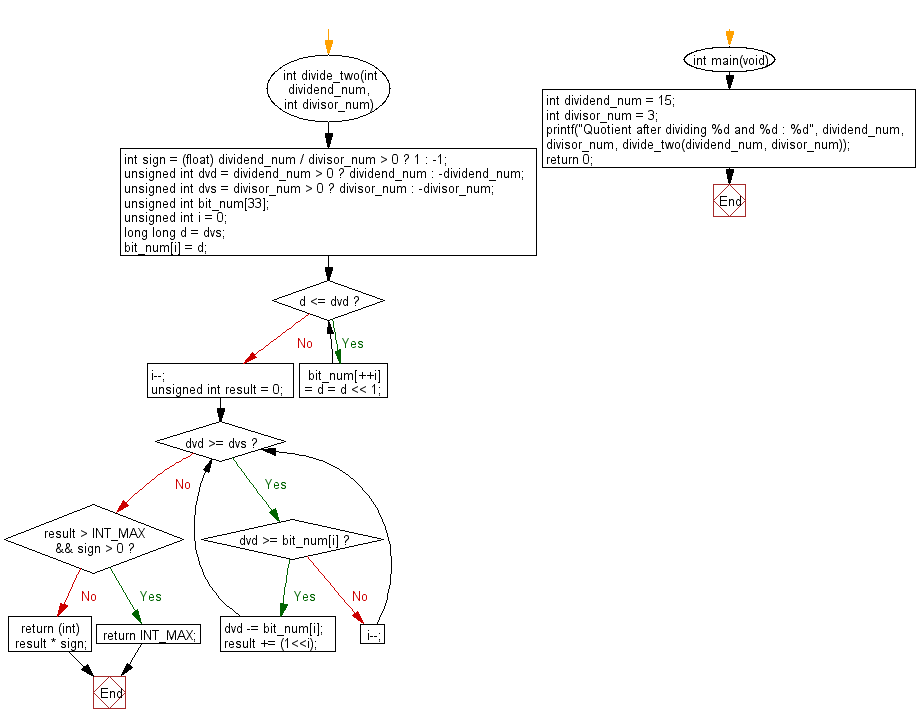﻿ C : Divide two integers without multiply, divide, modulating# C Exercises: Divide two given integers without using multiplication, division and mod operator

## C Programming Practice: Exercise-15 with Solution

Write a C programming to divide two given integers without using multiplication, division and mod operator. Return the quotient after dividing.

C Code:

``````#include <stdio.h>
#include <stdlib.h>
#include <limits.h>

int divide_two(int dividend_num, int divisor_num)
{
int sign = (float) dividend_num / divisor_num > 0 ? 1 : -1;
unsigned int dvd = dividend_num > 0 ? dividend_num : -dividend_num;
unsigned int dvs = divisor_num > 0 ? divisor_num : -divisor_num;
unsigned int bit_num;
unsigned int i = 0;
long long d = dvs;

bit_num[i] = d;
while (d <= dvd) {
bit_num[++i] = d = d << 1;
}
i--;

unsigned int result = 0;
while (dvd >= dvs) {
if (dvd >= bit_num[i]) {
dvd -= bit_num[i];
result += (1<<i);
} else {
i--;
}
}

if (result > INT_MAX && sign > 0) {
return INT_MAX;
}
return (int) result * sign;
}

int main(void)
{
int dividend_num = 15;
int divisor_num = 3;
printf("Quotient after dividing %d and %d : %d", dividend_num, divisor_num, divide_two(dividend_num, divisor_num));
return 0;
}
``````

Sample Output:

```Quotient after dividing 15 and 3 : 5
```

Pictorial Presentation:Flowchart:## C Programming Code Editor:

What is the difficulty level of this exercise?

Test your Programming skills with w3resource's quiz.

﻿

## C Programming: Tips of the Day

Maximum value of int:

In C:

```#include <limits.h>
then use
int imin = INT_MIN; // minimum value
int imax = INT_MAX;```

or

```#include <float.h>

float fmin = FLT_MIN;  // minimum positive value
double dmin = DBL_MIN; // minimum positive value

float fmax = FLT_MAX;
double dmax = DBL_MAX;```

Ref : https://bit.ly/3fi8yk9